### What is Fluid Mechanics?

• First, What is a fluid?
• Three common states of matter are solid, liquid, and gas.
• A fluid is either a liquid or a gas.
• If surface effects are not present, flow behaves similarly in all common fluids, whether gases or liquids.
• Example - The Penn State Sea Lion
Students in the Penn State Mechanical Engineering Department have designed and built a human powered submarine, named the "Sea Lion" as part of a national contest. In the preliminary stages of the design, back in the early 1990's, some wind tunnel testing was done on various hull shapes and fin shapes. Since the submarine moves below any surface effects, it was perfectly valid to run these tests in a wind tunnel (using air as the working fluid) rather than in water (the actual fluid in which the submarine moves). As will be discussed in a later learning module, drag and lift measurements must, of course, be scaled properly according to the rules of dimensional analysis.
• Example - PSU Harrier experiments
Several years ago, Professor Cimbala had a research grant from NASA to study the interaction of the jet exhaust from a harrier aircraft with the ground, while the aircraft is in hover with a wind blowing. Model tests were conducted in both a wind tunnel and a water tunnel. It was perfectly valid to run these tests in either air or water, since there were no free surface effects to worry about. As will be discussed in a later learning module, the results in either case must be scaled properly according to the rules of dimensional analysis.
• Formal definition of a fluid - A fluid is a substance which deforms continuously under the application of a shear stress.
• Definition of stress - A stress is defined as a force per unit area, acting on an infinitesimal surface element.
• Stresses have both magnitude (force per unit area) and direction, and the direction is relative to the surface on which the stress acts.
• There are normal stresses and tangential stresses.
• Pressure is an example of a normal stress, and acts inward, toward the surface, and perpendicular to the surface.
• A shear stress is an example of a tangential stress, i.e. it acts along the surface, parallel to the surface. Friction due to fluid viscosity is the primary source of shear stresses in a fluid.
• One can construct a free body diagram of a little fluid particle to visualize both the normal and shear stresses acting on the body:
Free Body Diagram, Fluid Particle at Rest:Consider a tiny fluid element (a very small chunk of the fluid) in a case where the fluid is at rest (or moving at constant speed in a straight line). A fluid at rest can have only normal stresses, since a fluid at rest cannot resist a shear stress. In this case, the sum of all the forces must balance the weight of the fluid element. This condition is known as hydrostatics. Here, pressure is the only normal stress which exists. Pressure always acts positively inward. Obviously, the pressure at the bottom of the fluid element must be slightly larger than that at the top, in order for the total pressure force to balance the weight of the element. Meanwhile, the pressure at the right face must be equal to that on the left face, so that the sum of forces in the horizontal direction is zero.
[Note: This diagram is two-dimensional, but an actual fluid element is three-dimensional. Hence, the pressure on the front face must also balance that on the back face.]
Free Body Diagram, Fluid Particle in Motion: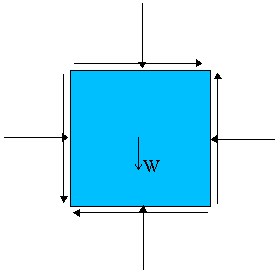Consider a tiny fluid element (a very small chunk of the fluid) that is moving around in some flow field. Since the fluid is in motion, it can have both normal and shear stresses, as shown by the free body diagram. The vector sum of all forces acting on the fluid element must equal the mass of the element times its acceleration (Newton's second law).
Likewise, the net moment about the center of the body can be obtained by summing the forces due to each shear stress times its moment arm. As the size of the fluid element shrinks to "zero," i.e. negligibly small, the shear stress acting on one face of the element must be the same magnitude as those acting on the other faces. Otherwise, there would be a net moment, causing the fluid element to spin rapidly!
[Note: To obtain force, one must multiply each stress by the surface area on which it acts, since stress is defined as force per unit area.]
• Definition of shear stress - Shear stress is defined as a force per unit area, acting parallel to an infinitesimal surface element.
• Shear stress is primarily caused by friction between fluid particles, due to fluid viscosity.
• Fluids at rest cannot resist a shear stress; in other words, when a shear stress is applied to a fluid at rest, the fluid will not remain at rest, but will move because of the shear stress.
• For a good illustration of this, consider the comparison of a fluid and a solid under application of a shear stress: A fluid can easily be distinguished from a solid by application of a shear stress, since, by definition, a fluid at rest cannot resist a shear stress.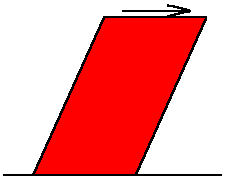If a shear stress is applied to the surface of a solid, the solid will deform a little, and then remain at rest (in its new distorted shape). One can say that the solid (at rest) is able to resist the shear stress.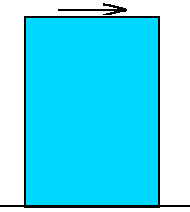Now consider a fluid (in a container). When a shear stress is applied to the surface of the fluid, the fluid will continuously deform, i.e. it will set up some kind of flow pattern inside the container. In other words, one can say that the fluid (at rest) is unable to resist the shear stress. That is to say, it cannot remain at rest under application of a shear stress.
• Another way of saying this is: A fluid at rest cannot resist a shear stress.
• Note, however, that a fluid at rest can resist a normal stress.
• Next, What is mechanics?
• The dictionary says mechanics is " ... the application of the laws of force and motion ... There are two branches, statics and dynamics. ..."
• So, putting it all together, fluid mechanics is the application of the laws of force and motion to fluids, i.e. liquids and gases. There are two branches of fluid mechanics:
• Fluid Statics or hydrostatics is the study of fluids at rest. The main equation required for this is Newton's second law for nonaccelerating bodies, i.e.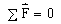.
• Fluid Dynamics is the study of fluids in motion. The main equation required for this is Newton's second law for accelerating bodies, i.e.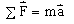.